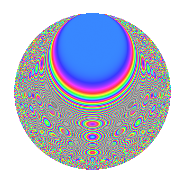# Properties

 Label 287.2.uLevel 287 Weight 2 Character orbit u Rep. character $$\chi_{287}(8,\cdot)$$ Character field $$\Q(\zeta_{20})$$ Dimension 160 Newforms 1 Sturm bound 56 Trace bound 0

# Related objects

## Defining parameters

 Level: $$N$$ = $$287 = 7 \cdot 41$$ Weight: $$k$$ = $$2$$ Character orbit: $$[\chi]$$ = 287.u (of order $$20$$ and degree $$8$$) Character conductor: $$\operatorname{cond}(\chi)$$ = $$41$$ Character field: $$\Q(\zeta_{20})$$ Newforms: $$1$$ Sturm bound: $$56$$ Trace bound: $$0$$

## Dimensions

The following table gives the dimensions of various subspaces of $$M_{2}(287, [\chi])$$.

Total New Old
Modular forms 240 160 80
Cusp forms 208 160 48
Eisenstein series 32 0 32

## Trace form

 $$160q - 4q^{3} + 36q^{4} - 28q^{6} + O(q^{10})$$ $$160q - 4q^{3} + 36q^{4} - 28q^{6} - 48q^{10} + 8q^{11} - 16q^{12} - 16q^{13} - 32q^{15} - 28q^{16} - 20q^{17} + 12q^{18} - 40q^{19} - 4q^{22} - 36q^{23} - 108q^{24} + 40q^{25} + 20q^{26} + 20q^{27} + 12q^{29} - 4q^{30} + 20q^{31} - 32q^{34} - 4q^{35} + 140q^{36} - 44q^{38} + 80q^{39} - 64q^{40} + 4q^{41} - 72q^{42} - 8q^{44} + 8q^{45} + 40q^{46} - 56q^{47} + 120q^{48} + 24q^{51} + 16q^{52} + 12q^{53} - 52q^{54} - 72q^{55} + 48q^{57} + 36q^{58} - 48q^{59} - 52q^{60} + 8q^{63} - 96q^{64} - 96q^{65} + 24q^{66} + 20q^{67} - 80q^{68} - 68q^{69} + 8q^{70} + 60q^{71} + 100q^{72} - 40q^{74} - 120q^{75} + 4q^{76} - 12q^{78} + 12q^{79} + 200q^{80} - 56q^{81} - 68q^{82} - 40q^{83} + 132q^{85} + 80q^{86} - 16q^{88} + 8q^{89} + 140q^{92} + 64q^{93} + 128q^{94} - 68q^{96} + 4q^{98} + 96q^{99} + O(q^{100})$$

## Decomposition of $$S_{2}^{\mathrm{new}}(287, [\chi])$$ into irreducible Hecke orbits

Label Dim. $$A$$ Field CM Traces $q$-expansion
$$a_2$$ $$a_3$$ $$a_5$$ $$a_7$$
287.2.u.a $$160$$ $$2.292$$ None $$0$$ $$-4$$ $$0$$ $$0$$

## Decomposition of $$S_{2}^{\mathrm{old}}(287, [\chi])$$ into lower level spaces

$$S_{2}^{\mathrm{old}}(287, [\chi]) \cong$$ $$S_{2}^{\mathrm{new}}(41, [\chi])$$$$^{\oplus 2}$$